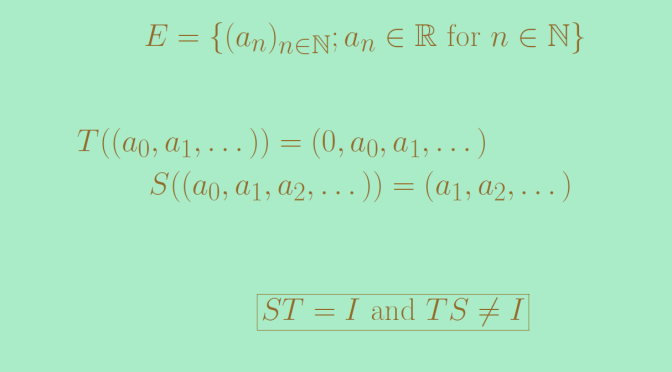# A linear map having a left inverse which is not a right inverse

We consider a vector space $$E$$ and a linear map $$T \in \mathcal{L}(E)$$ having a left inverse $$S$$ which means that $$S \circ T = S T =I$$ where $$I$$ is the identity map in $$E$$.

When $$E$$ is of finite dimension, $$S$$ is invertible.
For the proof we take $$x \in E$$. We have $$S(T(x)) = I(x) = x$$, which means that $$T(x)$$ is a pre-image of $$x$$ for $$S$$ proving that $$S$$ is onto. If $$E$$ is supposed to be of finite dimension, $$S$$ is also one-to-one and hence invertible and equal to $$T^{-1}$$. Another way to prove that $$S$$ is invertible is to use the determinant. We have $$\det(S T) = \det(S) \det(T)=\det(I)=1$$, hence $$\det(S) \neq 0$$ and $$S$$ is invertible.

What about the case where $$E$$ is of infinite dimension? In that case, a left inverse might not be a right inverse. We provide below a counterexample.

Consider the space $$E$$ of real sequences, the linear mapping $$T$$ that maps a sequence $$(a_0,a_1, \dots)$$ to the sequence $$(0,a_0,a_1, \dots)$$ and the linear mapping $$S$$ that maps a sequence $$(a_0,a_1,a_2, \dots)$$ to the sequence $$(a_1, a_2, \dots)$$. It is clear that $$ST = I$$. Now consider the sequence $$a=(1,0,0,0, \dots)$$. We have $$S(a)=\textbf{0}$$ where $$\textbf{0}$$ is the sequence that vanishes identically and also $$T S(a) = \textbf{0}$$ hence $$T S \neq I$$.